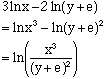Subject: logs Name: jessica Who is asking: Student Level: Secondary Question: Write as a single log 3ln x - 2ln(y+e) + 5ln(x-12) - 4ln y Hi Jessica, There are three properties of the logarithm functions that allow you to make the simplification required. They are log(ab) = log(a) + log(b) log(a/b) = log(a) - log(b) log(ab) = a log(b) I have written these in terms of log rather than ln since they are true for any logarithm. Looking at the first two terms of your problem, first apply property 3 and then property 2 to getNow try the rest of the problem. Cheers, Harley Go to Math Central